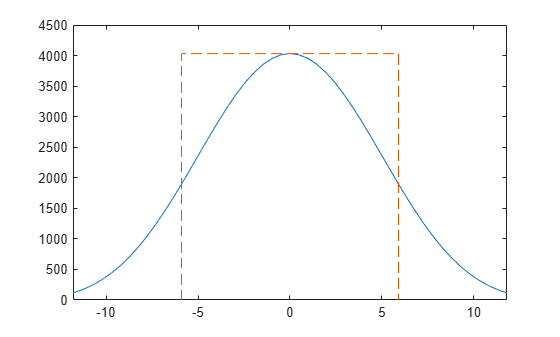# enbw

Equivalent noise bandwidth

## Syntax

``bw = enbw(window)``
``bw = enbw(window,fs)``

## Description

example

````bw = enbw(window)` returns the two-sided equivalent noise bandwidth, `bw`, for a uniformly sampled window, `window`. The equivalent noise bandwidth is normalized by the noise power per frequency bin.```

example

````bw = enbw(window,fs)` returns the two-sided equivalent noise bandwidth, `bw`, in Hz.```

## Examples

collapse all

Determine the equivalent noise bandwidth of a Hamming window 1000 samples in length.

`bw = enbw(hamming(1000))`
```bw = 1.3638 ```

Determine the equivalent noise bandwidth in Hz of a flat top window 10000 samples in length. The sample rate is 44.1 kHz.

`bw = enbw(flattopwin(10000),44.1e3)`
```bw = 16.6285 ```

Obtain the equivalent rectangular noise bandwidth of a Von Hann window and overlay the equivalent rectangular bandwidth on the window's magnitude spectrum. The window is 1000 samples in length and the sampling frequency is 10 kHz.

Set the sampling frequency, create the window, and obtain the discrete Fourier transform of the window with 0 frequency in the center of the spectrum.

```Fs = 10000; win = hann(1000); windft = fftshift(fft(win));```

Obtain the equivalent (rectangular) noise bandwidth of the Von Hann window.

`bw = enbw(hann(1000),Fs)`
```bw = 15.0150 ```

Plot the squared-magnitude DFT of the window and use the equivalent noise bandwidth to overlay the equivalent rectangle. The two-sided bandwidth is split evenly across the spectrum.

```freq = -(Fs/2):Fs/length(win):Fs/2-(Fs/length(win)); maxgain = 20*log10(abs(windft(length(win)/2+1))); plot(freq,20*log10(abs(windft))) hold on plot(bw/2*[-1 -1 1 1],[-40 maxgain maxgain -40],'--') hold off xlabel('Hz') ylabel('dB') axis([-60 60 -40 60])```## Input Arguments

collapse all

Uniformly sampled window vector, specified as a row or column vector with real-valued elements.

Example: `hamming(1000)`

Data Types: `double` | `single`

Sampling frequency, specified as a positive scalar.

## Output Arguments

collapse all

Equivalent noise bandwidth, specified as a positive scalar.

Data Types: `double` | `single`

collapse all

### Equivalent Noise Bandwidth

The equivalent noise bandwidth of a window is the width of a rectangle whose area contains the same total power as the window. The height of the rectangle is the peak squared magnitude of the window’s Fourier transform.

Assuming a sampling interval of 1, the total energy for the window, w(n), can be expressed in the frequency or time-domain as

`${\int }_{-1/2}^{1/2}{|W\left(f\right)|}^{2}df=\sum _{n}{|w\left(n\right)|}^{2}.$`

The peak magnitude of the window’s spectrum occurs at f = 0. This is given by

`${|W\left(0\right)|}^{2}={|\sum _{n}w\left(n\right)|}^{2}.$`

To find the width of the equivalent rectangular bandwidth, divide the area by the height.

`$\frac{{\int }_{-1/2}^{1/2}{|W\left(f\right)|}^{2}df}{{|W\left(0\right)|}^{2}}=\frac{\sum _{n}{|w\left(n\right)|}^{2}}{{|\sum _{n}w\left(n\right)|}^{2}}.$`

See Equivalent Rectangular Noise Bandwidth for an example that plots the equivalent rectangular bandwidth over the magnitude spectrum of a von Hann window.